SLUSAE5G August   2011  – April 2021

PRODUCTION DATA

1. Features
2. Applications
3. Description
4. Revision History
5. Pin Configuration and Functions
6. Specifications
7. Detailed Description
1. 7.1 Overview
2. 7.2 Functional Block Diagram
3. 7.3 Feature Description
4. 7.4 Device Functional Modes
8. Application and Implementation
1. 8.1 Application Information
2. 8.2 Typical Applications
1. 8.2.1 Typical Application Circuit Diagram with Ceramic Output Capacitors
2. 8.2.2 Typical Application Circuit
9. Power Supply Recommendations
10. 10Layout
11. 11Device and Documentation Support
12. 12Mechanical, Packaging, and Orderable Information

Package Options

Refer to the PDF data sheet for device specific package drawings

• DQP|22
• DQP|22
8.2.1.2.3 External Component Selection Using All Ceramic Output Capacitors

When a ceramic output capacitor is used, the stability criteria in Equation 7 cannot be satisfied. The ripple injection approach as shown in Figure 8-2 is implemented to increase the ripple on the VFB pin and make the system stable. In addition to the selections made using steps 1 through step 6 in Section 8.2.1.2.2, the ripple injection components must be selected. The C2 value can be fixed at 1 nF. The value of C1 can be selected between 10 nF to 200 nF.

Equation 11.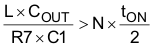where

• N is the coefficient to account for L and COUT variation

N is also used to provide enough margin for stability. It is recommended N=2 for VOUT ≤ 1.8 V and N=4 for VOUT ≥ 3.3 V or when L ≤ 250 nH. The higher VOUT needs a higher N value because the effective output capacitance is reduced significantly with higher DC bias. For example, a 6.3-V, 22-µF ceramic capacitor may have only 8 µF of effective capacitance when biased at 5 V.

Because the VFB pin voltage is regulated at the valley, the increased ripple on the VFB pin causes the increase of the VFB DC value. The AC ripple coupled to the VFB pin has two components, one coupled from SW node and the other coupled from the VOUT pin and they can be calculated using Equation 12 and Equation 13 when neglecting the output voltage ripple caused by equivalent series inductance (ESL).

Equation 12.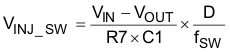Equation 13.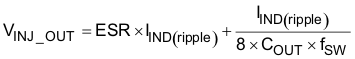It is recommended that VINJ_SW to be less than 50 mV and VINJ_TOTAL to be less than 60 mV. If the calculated VINJ_SW is higher than 50 mV, then other parameters need to be adjusted to reduce it. For example, COUT can be increased to satisfy Equation 11 with a higher R7 value, thereby reducing VINJ_SW. Use Equation 14 to calculate COUT capacitance needed. For a more holistic calculation, please reference the TPS53355 calculator on ti.com

Equation 14.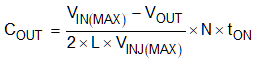The DC voltage at the VFB pin can be calculated by Equation 15:

Equation 15.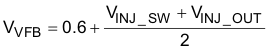And the resistor divider value can be determined by Equation 16:

Equation 16.